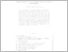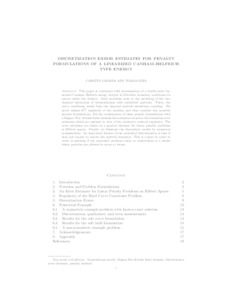Repository: Freie Universität Berlin, Math Department

# Discretization error estimates for penalty formulations of a linearized Canham-Helfrich-type energy

Gräser, C. and Kies, T. (2019) Discretization error estimates for penalty formulations of a linearized Canham-Helfrich-type energy. IMA Journal of Numerical Analysis, 39 (2). pp. 626-649. ISSN 0272-4979Preview

669kB

Official URL: https://doi.org/10.1093/imanum/drx071

## Abstract

This article is concerned with minimization of a fourth-order linearized Canham–Helfrich energy subject to Dirichlet boundary conditions on curves inside the domain. Such problems arise in the modeling of the mechanical interaction of biomembranes with embedded particles. There, the curve conditions result from the imposed particle–membrane coupling. We prove almost-H. 5. 2 regularity of the solution and then consider two possible penalty formulations. For the combination of these penalty formulations with a Bogner–Fox–Schmit finite element discretization, we prove discretization error estimates that are optimal in view of the solution’s reduced regularity. The error estimates are based on a general estimate for linear penalty problems in Hilbert spaces. Finally, we illustrate the theoretical results by numerical computations. An important feature of the presented discretization is that it does not require the particle boundary to be resolved. This is crucial to avoid re-meshing if the presented problem arises as a subproblem in a model where particles are allowed to move or rotate.

Item Type: Article Mathematical and Computer Sciences > Mathematics > Applied MathematicsMathematical and Computer Sciences > Mathematics > Numerical Analysis Department of Mathematics and Computer Science > Institute of Mathematics 2072 Silvia Hoemke 20 Apr 2017 09:27 19 Oct 2020 13:36

Repository Staff Only: item control page